﻿ Research on Particle Swarm Optimization Algorithm Based on Quantum Computing TechnologyPublications are Open
Access in this journal
Article Versions
Export Article
• Normal Style
• MLA Style
• APA Style
• Chicago Style
Research Article
Open Access Peer-reviewed

### Research on Particle Swarm Optimization Algorithm Based on Quantum Computing Technology

Guanghui Wang
American Journal of Electrical and Electronic Engineering. 2020, 8(1), 21-25. DOI: 10.12691/ajeee-8-1-3
Received November 03, 2019; Revised December 21, 2019; Accepted January 05, 2020

### Abstract

In view of the shortcomings of particle swarm optimization such as premature convergence to local optimization, particle swarm optimization algorithm based on quantum gate and particle swarm optimization algorithm based on quantum behavior are studied in this paper. The first algorithm uses the random observation method of quantum bit coding particles to simulate quantum particle collapse for generating a population and uses the quantum rotating gate to generate a new population. The adaptive mutation operator is used for ensuring the diversity of the population, effectively reducing the impact of local optimization; therefore, the robustness of the algorithm is improved. The second algorithm uses the probability density function of quantum computation to make the particles jump out of the local extreme points and fulfils the global search, which is more suitable for continuous optimization. The results of computational experiments show that both the two particle swarm optimization algorithms based on quantum technology have a good global convergence.

### 1. Introduction

The classical particle swarm optimization (PSO) algorithm is a random search algorithm based on swarm intelligence, which has the ability of global approximation, but because of its limited search space, it is easy to fall into local extremum. In 1996, Narayanan et al. 1 first combined quantum theory with evolutionary algorithm, proposed the concept of quantum genetic algorithm, and created a new direction of the integration of quantum computing and evolutionary algorithm. In 2000, Han Kuk Hyun 2 of South Korea introduced quantum chromosome coding based on quantum theory for the first time, using quantum gates to realize population renewal, etc. Compared with the traditional evolutionary algorithm, quantum evolutionary algorithm has the advantages of good population dispersion, strong global search ability, fast search speed, and easy to combine with other algorithms. Sun Jun et al. give a particle swarm optimization algorithm with quantum behavior, namely QPSO algorithm, in reference 3. The algorithm is simple and effective, with fast convergence speed, and the global search performance is much better than PSO algorithm. In quantum behavioral particle swarm optimization, the bound state particles described by probability density function can appear in any interval of the whole feasible search space with a certain probability, so the updated position can be much better than the current optimal position of the group, so as to meet the global convergence of the algorithm.

In this paper, particle swarm optimization algorithm based on quantum gate and particle swarm optimization algorithm based on quantum behavior are studied. The classical particle swarm optimization (PSO) algorithm is combined with quantum theory to encode particles using quantum bits, which is represented by the probability amplitude of particles. A quantum particle contains the information of several basic particle states. Based on the quantum superposition and quantum transition theory, a new population is generated by using quantum revolving gate. In addition, in the standard particle swarm optimization algorithm, through the mutual learning between groups and individuals, the search speed of particles is updated, and the probability density function of quantum computation is used to make particles jump out of the local extreme point to achieve global search. The computational results verify the stability and global convergence of the two particle swarm optimization algorithms based on quantum computing technology.

### 2. Particle Swarm Optimization Algorithm

In the particle swarm optimization (PSO) algorithm system, the potential solution of each optimization problem can be imagined as a point in search space, called "particle", and all particles have a fitness value determined by the objective function. Each particle flies at a certain speed in search space, which is dynamically adjusted according to its own flight experience and the flight experience of other particles. Generally, the particles will follow the best particles and search for the best solution through generation by generation. In each generation, the particle will track two best positions. The first is the best position the particle has found so far, which is called personal best (pbest) position. The other is the best position the whole particle swarm has found so far, which is called the global best (gbest) position. When the particles find the two extreme values, they update their speed and position according to the following formula: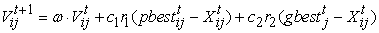(1)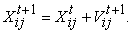(2)

Among them,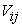is the speed of particle,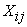is the current position of particle,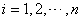,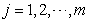, n is the size of population, m is the dimension of search space;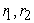is the random number between (0,1),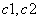are called learning factors, usually set as 2,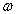is the inertia factor, mainly to weigh the ability of global search and local search. When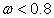, its local search ability is weak; when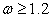, its global search ability. The ability is strong, generally taking the value between 0.1 and 0.9 4.

From the point of view of dynamics, the particle convergence process of particle swarm optimization algorithm is based on the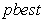point as the attractor. With the decrease of speed, the particle converges to the pbest point. Therefore, in the whole process, there is actually some form of attraction potential energy field at thepoint to attract particles, which is the reason why the whole particles can keep aggregation 5. However, in the classical PSO algorithm, particles fly along a certain trajectory in the classical mechanical state. The space of particle search is a limited area, so it cannot be guaranteed to find the global optimal solution.

### 3. Particle Swarm Optimization Algorithm Based on Quantum Gate

Quantum particle swarm optimization (QPSO) is a combination of quantum theory and particle swarm optimization (PSO). It takes advantage of the strength of quantum computing over classical computing, increases population diversity, and improves computing efficiency and performance to optimize its application in multimodal optimization. By using quantum probability amplitude coding and designing corresponding unitary matrix, the quantum update and movement are completed.

3.1. Population Initialization

The quantum evolutionary algorithm based on quantum gate is represented by Han et al. 2, which encodes every individual of the population with quantum bits. The quantum algorithm is interpreted as a probability algorithm, and the evolution and transfer of quantum states in quantum computation are realized by quantum gates. Particles are represented by quantum bits, which are called qubits. Qubits have two basic states, namely,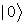state and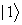state. At any time, the state of qubits can be represented by linear combination of basic states, which is called superposition state, namely: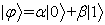, where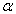and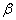are complex numbers, which is called probability amplitude. The superposition state can also be written as,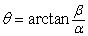is the phase of the qubit. During initialization, the particles are first initialized in the [0,1] interval, and then mapped into the domain space. The mapping relations is: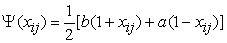(3)

This mapping is one-to-one mapping from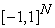to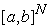.

The position of the particle is replaced by the quantum superposition state represented by the probability amplitude or phase. The space dimension is m, the population size is n, and the initialization state of the i-th particle is: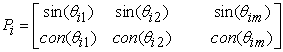(4)

where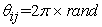is the quantum bit phase of the i-th particle in the j-th dimension,,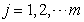. In addition, let the individual history optimum and global optimum of the i-th particle be: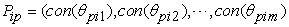(5)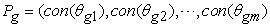(6)

This coding method enables a chromosome to represent the probability range of a ground state and expands the information capacity of the chromosome in the evolutionary algorithm. When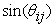or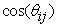tends to 0 or 1, quantum dyeing is proposed to collapse to a certain solution at an approximate rate. In addition, using trigonometric function to represent the probability amplitude of particles can effectively avoid the situation that particles cross the boundary and fall into local optimum.

3.2. Population Regeneration

In quantum computation, the transition between quantum states is realized by quantum energy, and the essence of quantum revolving gate is to change the size of the angle. The probability amplitude of qubit is updated by using quantum rotating gate as follows: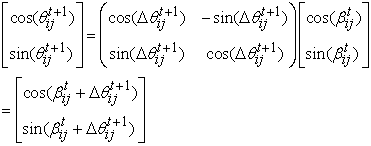(7)

where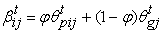is the angle of the local attractor. The rotation of quantum gate realizes the simultaneous movement of two positions by changing the quantum phase of particles, which improves the calculation efficiency of the algorithm. Therefore, the updated position of the i-th particle can be written as follows: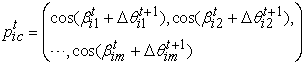(8)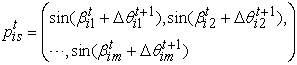(9)

where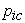is called cosine solution and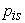is called sine solution. Since the sine and cosine are updated at the same time in each iteration, the search ergodicity of the solution space can be enhanced, and the optimization speed can be improved under the condition that the population size remains unchanged. At the same time, it can increase the number of global optimal solutions and improve the probability of global convergence. The calculation of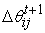is based on the following formula 3: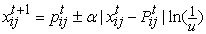(10)

where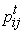is the local attractor,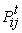is the individual historical optimum,is the control parameter, and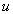is the random number evenly distributed between 0 and 1. From formula (10), we can get: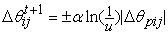(11)

where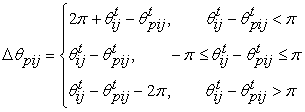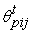is the best corresponding angle of particle history. Dynamic adjustment of the magnitude of the quantum rotating gate with the iterative process,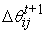depends on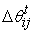size. The direction of the rotation angle is determined by u: when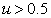, counter clockwise rotation (>0); when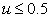, clockwise rotation (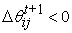). The control parameterchanges dynamically with the number of iterations. A simple linear reduction strategy is adopted: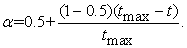(12)

where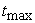is the maximum number of iterations.

Once the population falls into the trap of local optimization, the phase of particle renewal will soon tend to 0, and the population will hardly renew. In order to solve this problem, an adaptive probability is introduced that is defined as 6: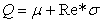(13)

where,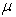and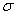are the variation adjustment parameters,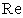is the optimal number of iterations without continuous or obvious update. If the population is continuously updated, the population will not be regulated. If the population is not smooth (will increase cumulatively), the probability of population regulation will increase.

3.4. Algorithm Flow

Step 1. Initialization population: set parameters.

Step 2. The first-generation population is initialized in the range of [0, 1].

Step 3. According to equation (4), the quantum state is expressed and the first fitness evaluation is carried out. The historical optimal value of particle is particle itself. The global optimal value of population is the best fitness value. If the jump condition is met, go to step 9; otherwise, go to step 4.

Step 4. Calculate the historical optimal phase of particles and global optimal phase of particles. Express the quantum state according to formula (4) and evaluate the fitness. Add 1 to the number of iterations. If the jump condition is met, go to step 9; otherwise, go to step 5.

Step 5. According to equation (11), the phase change of particles is updated and the particles are updated by equation (7) using quantum rotation gate.

Step 6. Judge whether to jump the particle's phase: if yes, execute formula (13); otherwise, go to Step7.

Step 7. According to the preset observation probability of particle state, the particle state is selected to collapse, and the particle is mapped to the solution space according to equation (3).

Step 8. Evaluate the fitness of the collapsed particles and decide whether the exit conditions are met: if yes, go to step 9; if no, update the global and historical optimal and go to step 4.

Step 9. Jump out of algorithm and finally output the best value.

### 4. Particle Swarm Optimization Based on Quantum Behavior

4.1. The Basic Evolution Equation of Particles

In the classical mechanical space, the particle's moving state has velocity and current state, which determines the prescribed trajectory. However, in quantum mechanics, the motion of particles is uncertain and there is no concept of trajectory. The quantum particle swarm optimization algorithm assumes that each particle has quantum behavior, and its state is described by the wave function. The probability distribution function of particle position is obtained by solving the steady Schrodinger equation, and then the position of particle motion in one-dimensional potential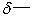potential trap with the attractor P-point as the center is simulated by inverse transformation method as follows 3: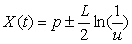(14)

where u is the uniformly distributed random function on the interval (0,1), i.e.,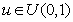; L is the characteristic length ofpotential trap, generally defined as: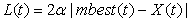(15)

where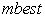is the average optimal position, which is the center of the optimal position of all particles. It can be calculated by the following formula: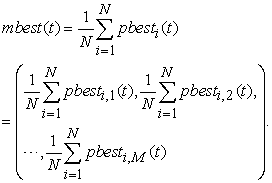(16)

According to formula (14) and (15), the renewal equation of particle position can be obtained as follows: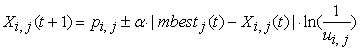(17)

where we use the local optimum of particles instead of attractors, that is,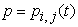, which is determined by the following formula in reference 7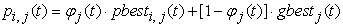(18)

where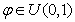,represents the average best position of all particles;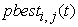represents the j-dimensional coordinate value of the current best position of the i-th particle in the t-th iteration;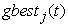represents the j-dimensional coordinate of the global best position in the t-th iteration;is the contraction-expansion coefficient. The update mode of the current optimal position and the global optimal position of particles is exactly the same as that of the corresponding parameters in the standard particle swarm optimization algorithm.

4.2. Dynamic Decreasing Strategy of ParameterIn order to have a large search space in the initial stage, the parametershould be relatively large, which can prevent the particle swarm from falling into the local optimum. With the increase of the number of iterations, the value ofshould be gradually reduced so that the particle swarm can search for the optimum in a small range, so as to approach the local optimum faster. Here, the logistic function curve is used to approximate the value of8: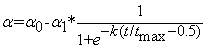(19)

where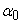and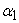represent the maximum and minimum value of control parameterrespectively;represents the maximum number of iterations of the algorithm; K is the curve smoothing factor, reflecting the smoothness degree of the curve, which determines the speed ofchange. Figure 1 shows the change of parameterin different iteration stages.

• Figure 1. Nonlinear decline curve based on logistic function
4.3. Algorithm Flow

Step 1. Initialize the position of particles in the particle swarm in the problem space.

Step 2. The average optimal position of particle swarm is calculated using equation (15).

Step 3. Calculate the fitness value of the particle's current position.

Step 4. Calculate the current global optimal position of the population.

Step 5. If the current global optimal position is better than that of the previous iteration, the global optimal position of the population is updated to its value.

Step 6. The positions of random points and the new positions of particles are calculated according to (17), (18) respectively.

Step 7. Judge whether the algorithm end condition is met: if not, return to step 2; otherwise, end the calculation and output the result.

### 5. Numerical Test and Analysis

In order to verify the effectiveness and optimization effect of the algorithm, this paper uses MATLAB 7.0 platform for numerical simulation, and uses Schaffer function of 6 proposed by J. D. Schaffer as the test function. Particle swarm optimization algorithm based on quantum logic gate and particle swarm optimization algorithm based on quantum behavior are used for comparative analysis.

Set population number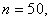The maximum number of iterations is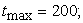c1=2, c2=2, Q=0.95, and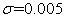.The dimension of space is m = 2. Schaffer function optimization problem is: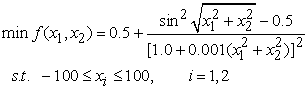Its global minimal is (0, 0), and there are many local minima in the range of about 3.13 from the global minima. The image of this function is shown in Figure 2.

• Figure 2. Schaffer function image
• Figure 3. Cross section of Schaffer function image
• Figure 4. Comparison of optimization results of Schaffer function

It can be seen from the cross-section Figure 3 of Figure 2 that there are multiple cycles of local extremum points around the global minimum point (0, 0). Experiments show that with the general particle swarm optimization algorithm, due to the continuous change of the random initial value, with the increase of the number of iterations, the extremum points show a jump change near (0, 0), and the accuracy of the local extremum converging to the global minimum value 0 is very unstable. Therefore, it is difficult to get the optimal result of Shaffer function with standard PSO. Figure 4 shows the comparison of the results of Schaffer function optimization based on quantum gate particle swarm optimization and quantum behavior particle swarm optimization.

### 6. Conclusion

It is obvious that the convergence speed and the accuracy of the optimization results of the particle swarm optimization based on quantum behavior are better than that of the particle swarm optimization based on quantum gate because of the introduction of the average best position in the algorithm. There is a waiting effect between particles, which greatly improves the ability of particle swarm to work together, thus enhancing the global search ability of the algorithm. The particle swarm optimization algorithm based on quantum gate uses quantum coding technology, through the update of quantum gate, generate the next generation population with better performance and with a larger probability.

On the other hand, due to the introduction of adaptive mutation operator in the iterative process of population renewal, it can effectively reduce the impact of local optimization and make up for the defects of premature convergence and poor local search ability of standard particle swarm optimization. Due to the quantum computing technology, the two particle swarm optimization algorithms studied in this paper have good global convergence.

### Fund

This research was supported by the National Natural Science Foundation (NSF) of China (No. 91437112).

### References

  Narayanan A, Moore M, "Quantum-inspired genetic algorithm," International Conference on Evolutionary Computation. IEEE, 1996, 61-66. In article  Han K H, Kim J H, "Genetic quantum algorithm and its application to combinational optimization problem," Proceedings of the International Congress on Evolutionary Computation. IEEE Press. 2000, 1354-1360. In article  Jun Sun, Bin Feng, WenboXu. "Particle swarm optimization with particles having quantum behavior," Congress on Evolutionary Computation，2004, 1354-1360. In article  Shi Y. H., Eberhart R. C., "Parameter selection in particle swarm optimization," Lecture Notes in Computer Science, V10 (1447), 1998, 591-600. In article View Article  Schaffer J. D, D. Garuana R A, Eshelman L J, Das R, "A study of control par-ameters affecting online performance of genetic algorithm for function optimization," In , 1993, 573-580. In article  Xu Bo, "A novel quantum continuous particle swarm optimization algorithm," Value Engineering, 2011, No.1 181-182. In article  Clerc, M.; Kennedy, J, "The particle swarm-explosion, stability, and convergence in a multidimensional complex space," IEEE Trans. Evolut. Comput. 2002(6), 58-73. In article View Article  TAO Chongyang, YANG Xinyu, YU Xiangshen, ZHAO Hang, "Control parameter analysis of quantum behaved particle swarm optimization algorithm," Journal of Computer pplication, 34(s2), 2014, 169-171. In articleThis work is licensed under a Creative Commons Attribution 4.0 International License. To view a copy of this license, visit http://creativecommons.org/licenses/by/4.0/